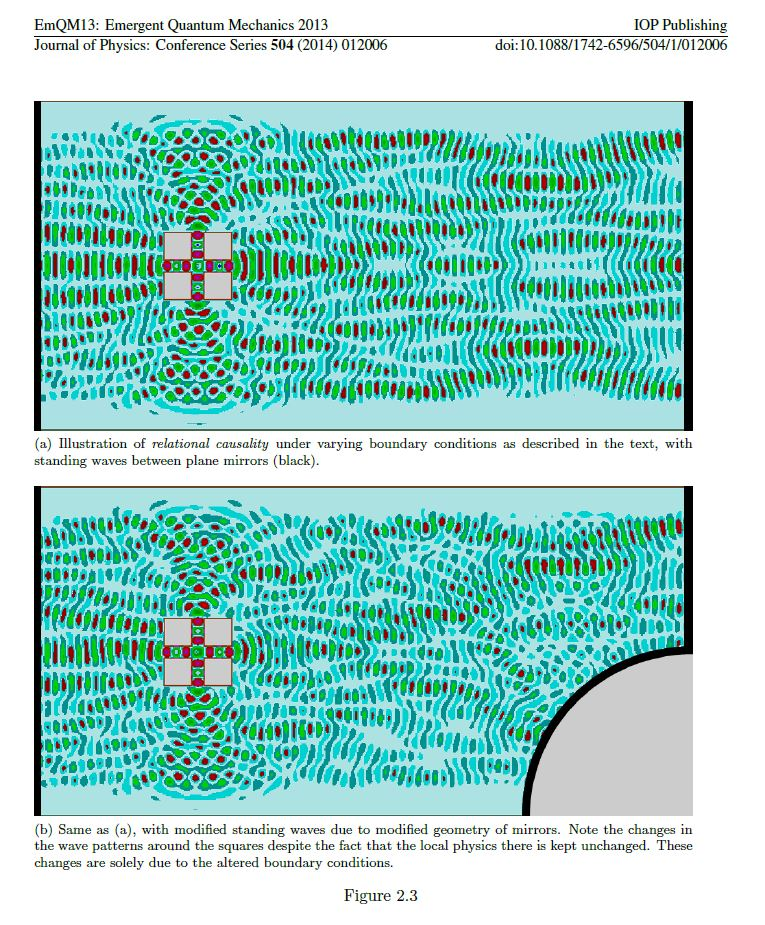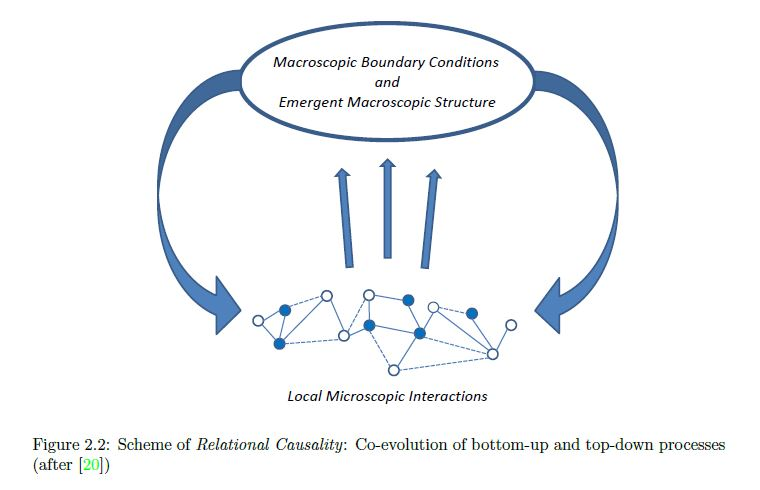Grössing, G., Fussy, S., Pascasio, J. M., & Schwabl, H. (2014, April). Relational causality and classical probability: Grounding quantum phenomenology in a superclassical theory. In Journal of Physics: Conference Series (Vol. 504, No. 1, p. 012006). IOP Publishing.

Abstract. : By introducing the concepts of \superclassicality” and \relational causality”, it is shown here that the velocity eld emerging from an n-slit system can be calculated as an
average classical velocity eld with suitable weightings per channel. No deviation from classical probability theory is necessary in order to arrive at the resulting probability distributions.
In addition, we can directly show that when translating the thus obtained expression for said velocity eld into a more familiar quantum language, one immediately derives the basic
postulate of the de Broglie-Bohm theory, i.e. the guidance equation, and, as a corollary, the exact expression for the quantum mechanical probability density current. Some other direct
consequences of this result will be discussed, such as an explanation of Born’s rule and Sorkin’s first and higher order sum rules, respectively.

http://iopscience.iop.org/1742-6596/504/1/012006/pdf/1742-6596_504_1_012006.pdf

classical probability theory

n-slit system

guidance equation